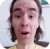FreeKB - Perl Hash (sort keys)
Perl - Hash (sort keys)Let's say you have the following hash. In this example, the hash has three keys (employee_key, id_key, department_key).

``````my %hash;
\$hash{employee_key}   = "John Doe";
\$hash{id_key}         = "123456";
\$hash{department_key} = "Engineering";``````

The following can be done to sort the keys alphabetically within the foreach loop.

This will not actually sort or modify the hash. This only sorts the keys as part of the foreach loop

``````foreach my \$key (sort keys %hash) {
print "\$key \n";
}``````

Which should return the following.

``````department
employee
id``````

Dumper

Dumper can be used to display the structure of the hash.

``````use Data::Dumper;
print Dumper \%hash;``````

Which should return the following. In this example, the key's are not sorted alphabetically.

``````\$VAR1 = {
'employee_key' => 'John Doe',
'id_key' => '123456',
'department_key' => 'Engineering'
};``````

The following can be done to sort the keys alphabetically.

This will not actually sort or modify the hash. This only sorts the keys for the Dumper output.

``\$Data::Dumper::Sortkeys = sub { [ sort keys %hash ] };``

Printing the hash . . .

``print Dumper \%hash;``

. . . should return the following. Now, the keys are sorted alphabetically.

``````\$VAR1 = {
'department_key' => 'Engineering',
'employee_key' => 'John Doe',
'id_key' => '123456'
};``````

We will never share your name or email with anyone. Enter your email if you would like to be notified when we respond to your comment.

Please enter 57607 in the box below so that we can be sure you are a human.

Web design by yours truely - me, myself, and I   |   jeremy.canfield@freekb.net   |